Next: Neural one-unit learning rules Up: Algorithms for ICA Previous: Algorithms for maximum likelihood

## Non-linear PCA algorithms

Nonlinear extensions of the well-known neural PCA algorithms [110,114,111] were developed in . For example, in , the following non-linear version of a hierarchical PCA learning rule was introduced: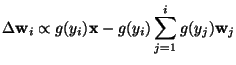(38)

where g is a suitable non-linear scalar function. The symmetric versions of the learning rules in [114,111] can be extended for the non-linear case in the same manner. In , a connection between these algorithms and non-linear versions of PCA criteria (see Section 4.3.4) were proven. In general, the introduction of non-linearities means that the learning rule uses higher-order information in the learning. Thus, the learning rules may perform something more related to the higher-order representation techniques (projection pursuit, blind deconvolution, ICA). In [84,112], it was proven that for well-chosen non-linearities, the learning rule in (38) does indeed perform ICA, if the data is sphered (whitened). Algorithms for exactly maximizing the nonlinear PCA criteria were introduced in .

An interesting simplification of the non-linear PCA algorithms is the bigradient algorithm . The feedback term in the learning rule (38) is here replaced by a much simpler one, giving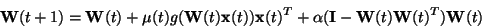(39)

where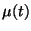is the learning rate (step size) sequence,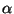is a constant on the range [.5,1], the function g is applied separately on every component of the vector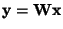, and the data is assumed to be sphered. A hierarchical version of the bigradient algorithm is also possible. Due to the simplicity of the bigradient algorithm, its properties can be analyzed in more detail, as in  and .Next: Neural one-unit learning rules Up: Algorithms for ICA Previous: Algorithms for maximum likelihood
Aapo Hyvarinen
1999-04-23